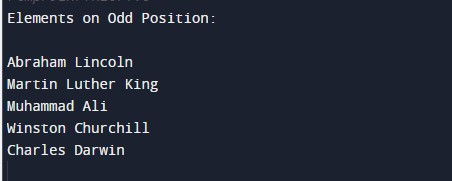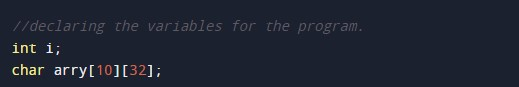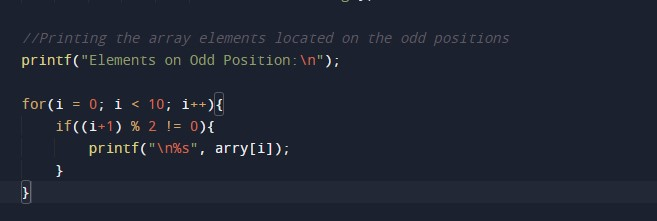C Program to Print Array Elements at Odd Position

In this tutorial you will learn about the C Program to Print Array Elements at Odd Position and its application with practical example.

C Program to Print Array Elements at Odd Position

In this tutorial, we will learn to create a C program that will Print Array Elements at Odd Positions in C programming.

Prerequisites

Before starting with this tutorial we assume that you are best aware of the following C programming topics:

• Operators in C Programming.
• Basic Input and Output function in C Programming.
• Basic C programming.
• For loop in C programming.
• Conditional Statements in C programming.
• Arithmetic Operators in C programming.

Print Array Elements at Odd Positions

As we all know array is a collection of similar data type elements. In an array, only one variable is declared which can store multiple values. First will take the number of elements of an array from the user. Then will take the elements from the user for the array. And at last, we will Print Array Elements at Odd Positions C Programming Language.

With the help of this program, we can Print Array Elements at Odd Positions.

Output:-In the above program, we have first initialized the required variable.• arry = it will hold the string value.
• i = it will hold the integer value for controlling the loop.

Insert the elements in the array for printing the odd index values.Printing the array elements of the odd index values in the output.In this tutorial we have learn about the C Program to Print Array Elements at Odd Position and its application with practical example. I hope you will like this tutorial.